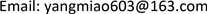﻿ 影响留守学生成绩因素的统计方法 Statistical Analysis of the Factors about Academic Performance of the Left-Behind Children

Vol.06 No.06(2017), Article ID:22217,6 pages
10.12677/AAM.2017.66094

Statistical Analysis of the Factors about Academic Performance of the Left-Behind Children

Miao Yang

L.A.S. Department, Chengdu College of University of Electronic Science and Technology of China, Chengdu SichuanReceived: Sep. 8th, 2017; accepted: Sep. 22nd, 2017; published: Sep. 28th, 2017ABSTRACT

The children whose parents work outside the home and left them at home, we call them the left-behind children. They are taken care of by grandparents or other relatives. We use contingency table methods and SPSS statistical software to analyze data. We can find the influential factors: Father’s occupation and the number of calls per week with parents.

Keywords:The Left-Behind Children, Academic Factor, Contingency Table1. 引言

2. 列联表简介

$H:{p}_{ij}={p}_{i\cdot }.{p}_{\cdot j},\text{\hspace{0.17em}}i=1,2,\cdots ,I,\text{\hspace{0.17em}}j=1,2,\cdots ,J$ .

${\chi }^{2}=\sum _{i=1}^{I}\sum _{j=1}^{J}\frac{{\left[{n}_{ij}-n\left(\frac{{n}_{i\cdot }}{n}\right)\left(\frac{{n}_{\cdot j}}{n}\right)\right]}^{2}}{n\left(\frac{{n}_{i\cdot }}{n}\right)\left(\frac{{n}_{\cdot j}}{n}\right)}=\sum _{i=1}^{I}\sum _{j=1}^{J}\frac{{\left[n{n}_{ij}-{n}_{i\cdot }{n}_{\cdot j}\right]}^{2}}{n{n}_{i\cdot }{n}_{\cdot j}}$

$P值=P\left\{{\chi }^{2}\left(\left(I-1\right)\left(J-1\right)\right)>{\chi }^{2}\right\}$

$I=J=2$ 时，列联表中只有4个格子，称为“四格表”，这时Pearson ${\chi }^{2}$ 统计量计算式可简单化为

${\chi }^{2}=\frac{n{\left({n}_{11}{n}_{22}-{n}_{12}{n}_{21}\right)}^{2}}{{n}_{1\cdot }{n}_{2\cdot }{n}_{\cdot 1}{n}_{\cdot 2}}$

3. 列联表分析影响因子

3.1. 父亲文化程度与成绩表现

3.2. 母亲文化程度与成绩表现

3.3. 父亲职业与成绩表现Table 2. Contingence table of fathers’ educational level and student performance on testsTable 3. Chi-square test table of fathers’ educational level and student performance on testsTable 4. Contingence table of mothers’ educational level and student performance on testsTable 5. Chi-square test table of mothers’ educational level and student performance on tests

3.4. 母亲职业与成绩表现

3.5. 和父母每个星期通话次数与成绩表现Table 6. Contingence table of fathers’ occupation and student performance on testsTable 7. Chi-square test table of fathers’ occupation and student performance on testsTable 8. Contingence table of mothers’ occupation and student performance on testsTable 9. Chi-square test table of mothers’ occupation and student performance on testsTable 10. Contingence table of number of calls per week with parents and student performance on testsTable 11. Chi-square test table of number of calls per week with parents and student performance on tests

4. 结束语

Statistical Analysis of the Factors about Academic Performance of the Left-Behind Children[J]. 应用数学进展, 2017, 06(06): 781-786. http://dx.doi.org/10.12677/AAM.2017.66094

1. 1. 一张. 留守儿童[J]. 瞭望新闻周刊, 1994(45): 36.

2. 2. 张志英. “留守幼儿”的孤僻心理[J]. 健康心理学杂志, 1998(1): 106-107.

3. 3. 谢西金, 杜屏. 家庭背景对初中生学习成绩影响的实证研究——以北京市11所中学调研为例[J]. 教育学术月刊, 2009(1): 50-52.

4. 4. 刘笑飞, 卢珂. 学生学业成绩与家庭社会经济背景相关性研究[J]. 天中学刊, 2009, 24(1): 130-133.

5. 5. 张绘, 龚欣, 尧浩根. 流动儿童学业表现及影响因素分析——来自北京的调研证据[J]. 北京大学教育评论, 2011, 9(3): 121-136.

6. 6. 赵必华. 影响城乡学生学习成绩差异的家庭因素及作用机制[J]. 中国人民大学教育学刊, 2011(4): 34-147.

7. 7. 蔡鼎文. 培养人才要从幼儿抓起——关于早期教育问题的探讨[J]. 江西师院学报, 1979(4): 86-90.

8. 8. 黄佳芬, 毛斐文. 家庭与学生学习的关系[J]. 上海师范大学学报(哲学社会科学版), 1984(2): 136-141.

9. 9. 李俊扬. 父母文化程度对学生数学学习成绩的影响初探[J]. 贵州师范大学学报(自然科学版), 1989(2): 92-95.

10. 10. 史希来. 属性数据分析引论[M]. 北京: 北京大学出版社, 2006.